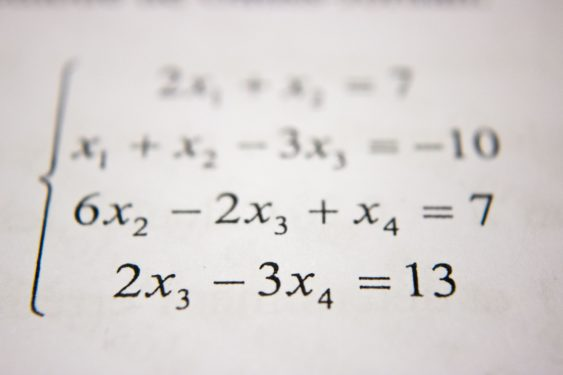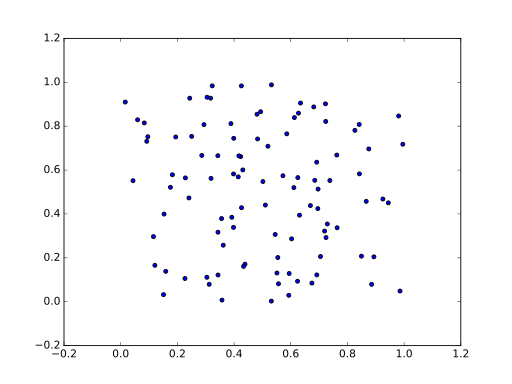# SAT Math Topics: 5 Tips on What You Need to Know Before You Go

Want to know what are the new SAT math topics? The math section of the SAT is designed to test your knowledge on problem solving, critical thinking, modeling, algebraic functions and using tools strategically. Seems like pretty broad concepts doesn’t it? Don’t worry, this is your definitive guide on the exact SAT math review topics that are currently being covered. By the end of this article you will know what math is on the new SAT, and how to review for it effectively!

##SAT Math Review

The SAT math topics are about getting real. The good news is that the SAT doesn’t test you on every single math topic you have ever had studied. Feel free to breathe a sight of relief. Instead, it selects areas deemed the most relevant in college courses and careers.

The SAT Math test focuses on areas that play a large role in colleges and a variety of careers, therefore applying real world math. These focus areas are:

• Heart of Algebra – create, manipulate and solve algebraic equations. These questions center around linear equations – equations that involve two variables that change as stated by a consistent pattern.
• Problem Solving and Data Analysis – is all about being quantitatively literate. These questions require you to create and use a model, as well as understanding the difference between the model predictions and actual data collected.
• Passport to Advance Math – includes questions that require the manipulation of complex equations and functions that are typically needed in STEM-based careers.

On top of these areas, the test also covers additional key concepts that don’t fall into any of the above categories. These include coordinate geometry, basic trigonometry, area and volume.

## 5 Ways to Review the SAT Math Topics

### 1. Know how to complete grid in questions

Students are often given advice to learn the style of questions and the format of the test. But what does this mean when it comes to the SAT?  In the SAT math section there are multiple choice questions and grid-in questions. Answering multiple choice questions are fairly straightforward – simply select the answer you think is correct.

###2. Become familiar with the provided formula sheet

Many students breathe a sigh of relief when they hear they don’t have to learn every single formula. But that doesn’t mean you can rest easy. You still need to know when to use each formula. Even knowing where the formulas are located on the sheet will save you valuable time. Have the sheet available during every single revision session and refer to it regularly. Pin it somewhere for you to study on a regular basis – a mirror, the refrigerator, or on the back of the bathroom door!

### 3. Work backwards

Sometimes it’s easier to start by looking at the multiple choice answers available. You may be lucky enough to rule out a couple of options straight away, but if not, try putting the various options into the equation. This tactic has potential to actually be more time consuming, so may not be ideal for every question, but it’s certainly a good one to have in your bag of tricks.

### 4. Use the daily practice app

The College Board not only provides official practice tests, but also an app to help track your progress. You will receive a question each day with hints and explanations. It’s a great way to maintain your math skills and to remind you to keep preparing for your math SAT!

### 5. Make the most of other subjects

It may come as no surprise that you use math skills in a variety of subjects. Because the SAT math section has such a focus on real world math, subjects such as science and social science are incredibly relevant. Apply your math knowledge in these subjects and you are unknowingly preparing yourself for the SAT math test. Any questions that involve data analysis, graphs, percentages, ratios and tables are useful in preparation for the SAT.

## Topics Covered in the New Math SAT

As you have already learned, the math SAT covers three main topics, with anything leftover categorized under additional math. This section will give you a SAT math topics breakdown, providing you with a thorough understanding of exactly what math is on the SAT.

### Heart of Algebra

In this section, questions are focused around equations based on real-world topics such as distance, speed, mass, volume or everyday financial topics.

• Solve linear equations and linear inequalities
• Interpret linear functions
• Graph linear equations
• Solve linear function word problems
• Solve systems of linear equations
• Answering these questions may require you to;
• Use multiple steps to simplify an expression or equation
• Select a graph that shows an algebraic equation that you saw in Algebra 1 course or Algebra 2. or choose the equation that describes a graph
• Indicate how a graph would be affected by a change in its equation

###Problem Solving and Data Analysis

In this section, questions revolve around the application of ratios, percentages, and proportional reasoning. We’ve come up with a collection of more topics and what might be asked of you below:

• Ratios, rates, percentages, units, and proportions – solve multi-step problems with a given ratio, rate, percentage or unit. You will also need to know how to calculate ratios, rates, percentages and units using the information provided.
• Table data and data inferences– analyze the data presented on a table and use it to answer questions
• Scatterplots – select the best equation to fit various scatterplots.
• Graphs and tables – understand and identify key features, as well as summarizing and evaluating the data presented in them
• Data collection and conclusions – determine whether data collection methods are accurate and reliable
• Statistics – determine mean, median, mode, range, and/or standard deviationStudents who are interested in STEM-based careers will need to pay particular attention to these type of questions. They involve complex equations and functions, and focus on the following areas;

• Interpreting nonlinear expressions
• Quadratic and exponential word problems
• Operations with rational expressions and polynomials
• Polynomial factors and graphs
• Nonlinear equation graphs
• Structure in expressions
• Isolating quantities
• Functions

Now that you are familiar with the new SAT math topics you can go ahead and prepare easily and aim to get the results that you need for schools! Always remember that the SAT math exam is only covering information that you’ve already learned.  So prepping and taking some time to review the material will help to refresh your memory and remember how to quickly and accurately complete problems in these areas. Remember to work hard and apply yourself, the rest is easy!### Enjoy All The Benefits

You don’t pay your first hour unless you find it a good fit.

Only pay for the time you need.

No subscriptions or upfront payments.

Find Tutors Near You